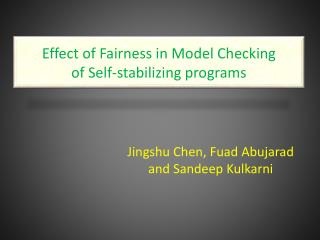Download PresentationEffect of Fairness in Model Checking of Self-stabilizing programs

# Effect of Fairness in Model Checking of Self-stabilizing programs

Download Presentation## Effect of Fairness in Model Checking of Self-stabilizing programs

- - - - - - - - - - - - - - - - - - - - - - - - - - - E N D - - - - - - - - - - - - - - - - - - - - - - - - - - -
##### Presentation Transcript

1. Effect of Fairness in Model Checking of Self-stabilizing programs Jingshu Chen, FuadAbujarad and SandeepKulkarni

2. Long term view

3. Outline • Problem Statement • Related work • Our approach • Results • Summary

4. Problem Statement • To verify self-stabilizing programs by symbolic model checking • stabilization: • ability of a system to converge in finite number of steps from arbitrary states to desired state. • complex algorithm • Symbolic model checking: doesn’t require the designer to have considerable experience in logic reasoning.

5. Case Study-K-state Program Two actions: • x0= xn -> x0=(x0+1) mod K • xi!=xi-1 ->xi=x(i-1) Note that: • the domain of x is [0,..,K-1] • This program is known to be self-stabilizing if K>N. In subsequent discussion, we let K=N+1. p0 p1 pn x0 p2 xn pn-1 p3 xi-1 xi pi-1 pi

6. Case Study-K-state Program Legal state: • For Process 0 either x=x[n] or x=(x[n]+1) mod K • For i=1.. N, either x[i-1]=x[i] or x[i-1]=x[i]+1 p0 p1 pn x0 p2 xn pn-1 p3 xi-1 xi pi-1 pi

7. Previous work • T’s work has demonstrate feasibility of applying symbolic model checking for verifying self-stabilizing programs. • The result shows that verification is feasible only for programs with a small number of process.

8. Approach(1) • Observation: - the current approach is done under weak fairness computation; - current model checker focus on weak fairness in representation of fairness. • Our approach is to verify self-stabilization under unfair computation.

9. Case Study- K-state program (k=3) Verification under weak fairness Two actions: • x0= xn -> x0=(x0+1) mod K • xi!=xi-1->xi=x(i-1)

10. Case Study- K-state program (k=3) Verification under unfair computation

11. Results(1) In spite of the improved hardware, the ability to verify self-stabilizing programs under weak fairness remains essentially the same. Scalability of verifying self-stabilization can be significantly improved for the case where the program is correct self-stabilizing without fairness.

12. Approach(2) • For the case where weak fairness is essential for self-stabilization, • Decomposition • Utilizing the weak version of stabilization-weak stabilization

13. Results(2)

14. Results(3) Verification of weak stabilization is substantially more scalable. This result also validates the suggestion in Gouda’s work that weak stabilization is easier to verify than self-stabilization.

15. Summary • If self-stabilization is possible without fairness then cost of verifying self-stabilization is substantially lower. • This is the first paper that has shown feasibility of verifying the typical self-stabilizing programs, e.g., K-state program, with large number of processes. • We also identify two approaches for those cases where weak fairness is essential for self-stabilization.